## RS Aggarwal Class 7 Solutions Chapter 6 Algebraic Expressions Ex 6C

These Solutions are part of RS Aggarwal Solutions Class 7. Here we have given RS Aggarwal Solutions Class 7 Chapter 6 Algebraic Expressions Ex 6C.

Other Exercises

Find each of the following products:
Question 1.
Solution:
4a(3a + 7b) = 4a x 3a + 4a x 7b = 12a2 + 28ab

Question 2.
Solution:
5a(6a – 3b) = 5a x 6a – 5a x 3b = 30a2 – 15ab

Question 3.
Solution:
8a(2a + 5b) = 8a2 x 2a + 8a2 x 5b = 16a3 + 40a2b

Question 4.
Solution:
9x2 (5x + 7) = 9x2 x 5x + 9x2 x 7 = 45x3 + 63x2

Question 5.
Solution:
ab(a2 – b2) = ab x a2 – ab x b2 = a3b – ab3

Question 6.
Solution:
2x2 (3x – 4x2) = 2x2 x 3x – 2x2 x 4x2 = 6x3 – 8x4

Question 7.
Solution: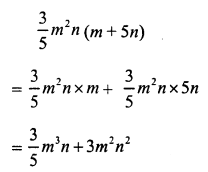Question 8.
Solution:
-1 7x2 (3x – 4) = -17x2 x 3x – 17x2 x (-4) = -51x3 + 68x2

Question 9.
Solution:Question 10.
Solution:
-4x2y (3x2 – 5y)
= -4xy x 3x2 – 4xy x (-5y)
= -12xy + 20xy2

Question 11.
Solution: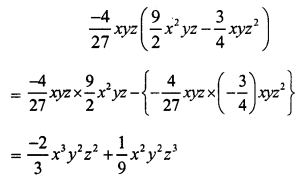Question 12.
Solution:
9t2 (t + 7t3) = 9t2 x t + 9t2 x 7t3 = 9t3 + 63t5

Question 13.
Solution: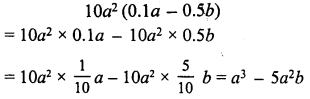Question 14.
Solution: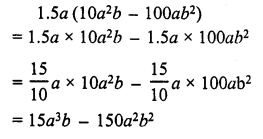Question 15.
Solution: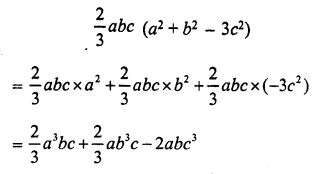Question 16.
Solution:
24x2 (1 – 2x)
= 24x2 x 1 – 24x2 x 2x
= 24x2 – 48x3
If x = 2, then
24x2 – 48x3
= 24(2)2 – 48(2)3
= 24 x 4 – 48 x 8
= 96 – 384
= -288

Question 17.
Solution: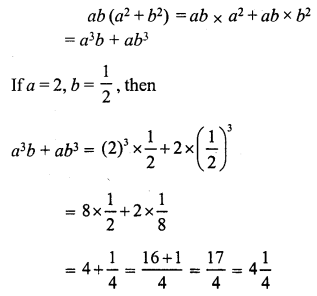Question 18.
Solution:
s (s2 – st) = s x s2 – s x st = s3 – s2t
If s = 2, t = 3, then
s3 – s2t = (2)3 – (2)2 x 3 = 8 – 4 x 3 = 8 – 12 = -4

Question 19.
Solution:
-3y (xy + y2) = -3y x xy + (-3y) x y2 = -3xy2 – 3y3
if x = 4, y = 5, then
-3xy2 – 3y3
= -3(4)(5)2 – 3(5)3 = -3 x 4 x 25 – 3 x 125 = -300 – 375 = -675

Simplify each of the following:
Question 20.
Solution:
a(b – c) + b(c – a) + c(a – b) = ab – ac + bc – ab + ac – bc = 0

Question 21.
Solution:
a(b – c) – b(c – a) – c(a – b) = ab – ac – bc + ab – ac + bc = 2ab – 2ac

Question 22.
Solution:
3x2 + 2(x + 2) – 3x (2x + 1)
= 3x2 + 2x + 4 – 6x2 – 3x = 3x2 – 6x2 + 2x – 3x + 4 = -3x2 – x + 4

Question 23.
Solution:
x (x + 4) + 3x (2x2 – 1) + 4x2 + 4
= x2 + 4x + 6x3 – 3x + 4x2 + 4
= 6x3 + x2 + 4x2 + 4x – 3x + 4
= 6x3 + 5x2 + x + 4

Question 24.
Solution:
2x2 + 3x (1 – 2x3) + x (x + 1)
= 2x2 + 3x – 6x4 + x2 + x
= – 6x4 + 2x2 + x2 + 3x + x
= – 6x4 + 3x2 + 4x

Question 25.
Solution:
a2b (a – b2) + ab(4ab – 2a2) – a3b (1 – 2b)
= a3b – a2b3 – 2a3b2 + 4a2b3 – 2a3b2 – a3b + 2a3b2
= a3b – a3b + 2a3b2 – a2b3 + 4a263
= 3a2b3

Question 26.
Solution:
4st (s – t) – 6s2 (t – t2) – 3t2 (2s2 – s) + 2st(s – t)
= 4s2t – 4st2 – 6s2t + 6s2t2 – 6s2t2 + 3st2 + 2s2t – 2st2
= 4s2t – 6s2t + 2s2t – 4st2 + 3st2 – 2st2 + 6s2t2 – 6s2t2
= 6s2t – 6s2t – 6st2 + 3st2 + 6s2t2 – 6s2t2
= – 3st2

Hope given RS Aggarwal Solutions Class 7 Chapter 6 Algebraic Expressions Ex 6C are helpful to complete your math homework.

If you have any doubts, please comment below. Learn Insta try to provide online math tutoring for you.